Updating search results...

# 18 Results

View
Selected filters:
• rectangles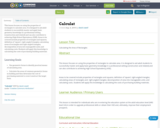Unrestricted Use
CC BY
Rating
0.0 stars

This lesson focuses on using the properties of rectangles to calculate area. It is designed to aid adult students to successfully master and apply basic geometry knowledge in a professional setting (construction and related) and can also contribute to achieving High School Equivalency (HSE).
Areas to be covered include properties of rectangles and squares; definition of ‘square’; right-angled triangles; calculating areas of rectangles and right-angled triangles; decomposition of area into manageable units; and calculating costs. Students will apply this knowledge to calculating the costs of purchasing building materials.

Subject:
Mathematics
Material Type:
Interactive
Lesson Plan
08/08/2019Unrestricted Use
CC BY
Rating
0.0 stars

This lesson focuses on calculating the areas of rectangles. It is designed to enable adult students to successfully master basic geometry knowledge in order to achieve their High School Equivalency (HSE). Areas to be covered include types of polygons, quadrilaterals, rectangles; calculating areas of rectangle and calculating costs. Students will apply this knowledge to practical areas of their lives such as calculating the costs of purchasing carpets or painting of walls

Subject:
Mathematics
Material Type:
Interactive
Lesson Plan
08/08/2019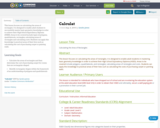Unrestricted Use
CC BY
Rating
0.0 stars

This lesson focuses on calculating the areas of rectangles. It is designed to enable adult students to successfully master basic geometry knowledge in order to achieve their High School Equivalency Diploma (HSED). Areas to be covered include types of polygons, quadrilaterals, rectangles; calculating areas of rectangles and calculating costs. Students can apply this knowledge to practical areas of their lives such as calculating the cost of purchasing carpet or painting walls.

Subject:
Mathematics
Material Type:
Interactive
Lesson Plan
08/08/2019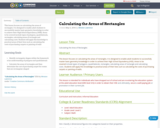Unrestricted Use
CC BY
Rating
0.0 stars

This lesson focuses on calculating the areas of rectangles. It is designed to enable adult students to successfully master basic geometry knowledge in order to achieve their High School Equivalency (HSE). Areas to be covered include types of polygons, quadrilaterals, rectangles; calculating areas of rectangle and calculating costs. Students will apply this knowledge to practical areas of their lives such as calculating the costs of purchasing carpets or painting of walls

Subject:
Mathematics
Material Type:
Interactive
Lesson Plan
05/05/2016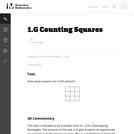Unrestricted Use
CC BY
Rating
0.0 stars

This challenging problem and brainteaser gives first graders an opportunity to compose and decompose squares.

Subject:
Geometry
Mathematics
Measurement and Data
Material Type:
Activity/Lab
Provider:
Illustrative Mathematics
Provider Set:
Illustrative Mathematics
Author:
Illustrative Mathematics
09/02/2012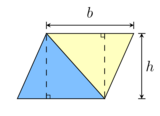Conditional Remix & Share Permitted
CC BY-SA
Rating
0.0 stars

Measurement in Grade 7 sees us move from simply finding the perimeter and area of a common object, such as a rectangle or triangle, and move into complex shapes that may involve a combination of different shapes or circles.&nbsp;This unit reviews the basics of finding the area of rectangles, triangles, and parallelograms. It concludes with some real-life problems to which these concepts can be applied.This unit is designed for the Alberta Grade 7 Curriculum.

Subject:
Measurement and Data
Material Type:
Activity/Lab
Homework/Assignment
Module
Unit of Study
Author:
10/10/2022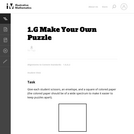Unrestricted Use
CC BY
Rating
0.0 stars

This instructional task gives students a hands-on experience with composing and decomposing geometric figures.

Subject:
Geometry
Mathematics
Measurement and Data
Material Type:
Activity/Lab
Provider:
Illustrative Mathematics
Provider Set:
Illustrative Mathematics
Author:
Illustrative Mathematics
09/09/2012Conditional Remix & Share Permitted
CC BY-NC
Rating
0.0 stars
Rating
0.0 stars
Subject:
Mathematics
Material Type:
Full Course
Provider:
Pearson
10/06/2016Conditional Remix & Share Permitted
CC BY-NC
Rating
0.0 stars

Expressions

Type of Unit: Concept

Prior Knowledge

Students should be able to:

Write and evaluate simple expressions that record calculations with numbers.
Use parentheses, brackets, or braces in numerical expressions and evaluate expressions with these symbols.
Interpret numerical expressions without evaluating them.

Lesson Flow

Students learn to write and evaluate numerical expressions involving the four basic arithmetic operations and whole-number exponents. In specific contexts, they create and interpret numerical expressions and evaluate them. Then students move on to algebraic expressions, in which letters stand for numbers. In specific contexts, students simplify algebraic expressions and evaluate them for given values of the variables. Students learn about and use the vocabulary of algebraic expressions. Then they identify equivalent expressions and apply properties of operations, such as the distributive property, to generate equivalent expressions. Finally, students use geometric models to explore greatest common factors and least common multiples.

Subject:
Algebra
Mathematics
Material Type:
Unit of Study
Provider:
Pearson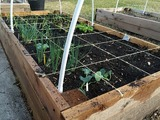Conditional Remix & Share Permitted
CC BY-NC
Rating
0.0 stars

Students use a rectangular area model to understand the distributive property. They watch a video to find how to express the area of a rectangle in two different ways. Then they find the area of rectangular garden plots in two ways.Key ConceptsThe distributive property can be used to rewrite an expression as an equivalent expression that is easier to work with. The distributive property states that multiplication distributes over addition.Applying multiplication to quantities that have been combined by addition: a(b + c)Applying multiplication to each quantity individually, and then adding the products together: ab + acThe distributive property can be represented with a geometric model. The area of this rectangle can be found in two ways: a(b + c) or ab + ac. The equality of these two expressions, a(b + c) = ab + ac, is the distributive property.Goals and Learning ObjectivesUse a geometric model to understand the distributive property.Write equivalent expressions using the distributive property.

Subject:
Algebra
Geometry
Material Type:
Lesson Plan
09/21/2015Conditional Remix & Share Permitted
CC BY-NC
Rating
0.0 stars

Four full-year digital course, built from the ground up and fully-aligned to the Common Core State Standards, for 7th grade Mathematics. Created using research-based approaches to teaching and learning, the Open Access Common Core Course for Mathematics&nbsp;is designed with student-centered learning in mind, including activities for students to develop valuable 21st century skills and academic mindset.

Subject:
Mathematics
Material Type:
Full Course
Provider:
Pearson
10/06/2016Conditional Remix & Share Permitted
CC BY-NC
Rating
0.0 stars

Constructions and Angles

Unit Overview

Type of Unit: Concept

Prior Knowledge

Students should be able to:

Use a protractor and ruler.
Identify different types of triangles and quadrilaterals and their characteristics.

Lesson Flow

After an initial exploratory lesson involving a paper folding activity that gets students thinking in general about angles and figures in a context, the unit is divided into two concept development sections. The first section focuses on types of angles—adjacent, supplementary, complementary, and vertical—and how they are manifested in quadrilaterals. The second section looks at triangles and their properties, including the angle sum, and how this affects other figures.

In the first set of conceptual lessons, students explore different types of angles and where the types of angles appear in quadrilaterals. Students fold paper and observe the angles formed, draw given angles, and explore interactive sketches that test many cases. Students use a protractor and ruler to draw parallelograms with given properties. They explore sketches of parallelograms with specific properties, such as perpendicular diagonals. After concluding the investigation of the angle types, students move on to the next set.

In the second set of conceptual development lessons, students focus on triangles. Students again fold paper to create figures and certain angles, such as complementary angles.

Students draw, using a protractor and ruler, other triangles with given properties. Students then explore triangles with certain known and unknown elements, such as the number of given sides and angles. This process starts with paper folding and drawing and continues with exploration of interactive sketches. Students draw conclusions about which cases allow 0, 1, 2, or an infinite number of triangles. In the course of the exploration, students discover that the sum of the measure of the interior angles of a triangle is 180°. They also learn that the sum of the measures of the interior angles of a quadrilateral is 360°. They explore other polygons to find their angle sum and determine if there is a relationship to angle sum of triangles. The exploration concludes with finding the measure of the interior angles of regular polygons and speculating about how this relates to a circle.

Lastly, students solve equations to find unknown angle measures. Using their previous experience, students find the remaining angle measures in a parallelogram when only one angle measure is given. Students also play a game similar to 20 Questions to identify types of triangles and quadrilaterals. Having completed the remaining lessons, students have a four-day Gallery to explore a variety of problems.

The unit ends with a unit assessment.

Subject:
Geometry
Mathematics
Material Type:
Unit of Study
Provider:
PearsonConditional Remix & Share Permitted
CC BY-NC
Rating
0.0 stars

Students learn how the diagonals of a rhombus are related. They use interactive sketches to learn about the properties of the angles and diagonals of squares, rectangles, rhombuses, parallelograms, and other quadrilaterals.Key ConceptsThe sum of the measures of the angles of all quadrilaterals is 360&deg;.The alternate angles (nonadjacent angles) of rhombuses and parallelograms have the same measure.The measure of the angles of rectangles and squares is 90&deg;.The consecutive angles of parallelograms and rhombuses are supplementary. This applies to squares and rectangles as well.The diagonals of a parallelogram bisect each other.The diagonals of a rectangle are congruent and bisect each other.The diagonals of a rhombus bisect each other and are perpendicular.Goals and Learning ObjectivesMeasure the angles formed by the intersection of the diagonals of a rhombus.Explore the relationships of the angles of different squares, rectangles, rhombuses, parallelograms, and other quadrilaterals.Explore the relationships of the diagonals of different squares, rectangles, rhombuses, parallelograms, and other quadrilaterals.

Subject:
Geometry
Material Type:
Lesson Plan
09/21/2015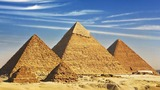Conditional Remix & Share Permitted
CC BY-NC
Rating
0.0 stars

Zooming In On Figures

Unit Overview

Type of Unit: Concept; Project

Length of Unit: 18 days and 5 days for project

Prior Knowledge

Students should be able to:

Find the area of triangles and special quadrilaterals.
Use nets composed of triangles and rectangles in order to find the surface area of solids.
Find the volume of right rectangular prisms.
Solve proportions.

Lesson Flow

After an initial exploratory lesson that gets students thinking in general about geometry and its application in real-world contexts, the unit is divided into two concept development sections: the first focuses on two-dimensional (2-D) figures and measures, and the second looks at three-dimensional (3-D) figures and measures.
The first set of conceptual lessons looks at 2-D figures and area and length calculations. Students explore finding the area of polygons by deconstructing them into known figures. This exploration will lead to looking at regular polygons and deriving a general formula. The general formula for polygons leads to the formula for the area of a circle. Students will also investigate the ratio of circumference to diameter ( pi ). All of this will be applied toward looking at scale and the way that length and area are affected. All the lessons noted above will feature examples of real-world contexts.
The second set of conceptual development lessons focuses on 3-D figures and surface area and volume calculations. Students will revisit nets to arrive at a general formula for finding the surface area of any right prism. Students will extend their knowledge of area of polygons to surface area calculations as well as a general formula for the volume of any right prism. Students will explore the 3-D surface that results from a plane slicing through a rectangular prism or pyramid. Students will also explore 3-D figures composed of cubes, finding the surface area and volume by looking at 3-D views.
The unit ends with a unit examination and project presentations.

Subject:
Geometry
Mathematics
Material Type:
Unit of Study
Provider:
PearsonConditional Remix & Share Permitted
CC BY-NC
Rating
0.0 stars

Students critique their work from the Self Check and redo the task after receiving feedback. Students then take a quiz to review the goals of the unit.Key ConceptsStudents understand how to find the area of figures such as rectangles and triangles. They have applied that knowledge to finding the area of composite figures and regular polygons. The area of regular polygons was extended to understand the area of a circle. Students also applied ratio and proportion to interpret scale drawings and redraw them at a different scale.GoalsCritique and revise student work.Apply skills learned in the unit.Understand two-dimensional measurements:Area of composite figures, including regular polygons.Area and circumference of circles.Interpret scale drawings and redraw them at a different scale.SWD: Make sure all students have the prerequisite skills for the activities in this lesson.Students should understand these domain-specific terms:composite figuresregular polygonsareacircumferencescale drawingstwo dimensionalIt may be helpful to preteach these terms to students with disabilities.ELL: As academic vocabulary is reviewed, be sure to repeat it and allow students to repeat after you as needed. Consider writing the words as they are being reviewed. Allow enough time for ELLs to check their dictionaries if they wish.

Subject:
Geometry
Material Type:
Lesson Plan
09/21/2015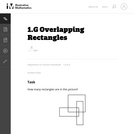Unrestricted Use
CC BY
Rating
0.0 stars

This challenging problem and brainteaser gives students an opportunity to compose and decompose polygons to make rectangles.

Subject:
Geometry
Mathematics
Measurement and Data
Material Type:
Activity/Lab
Provider:
Illustrative Mathematics
Provider Set:
Illustrative Mathematics
Author:
Illustrative Mathematics
08/29/2012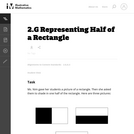Unrestricted Use
CC BY
Rating
0.0 stars

This task provides a context for indentifying different ways of representing half of a rectangle.

Subject:
Geometry
Mathematics
Ratios and Proportions
Material Type:
Activity/Lab
Provider:
Illustrative Mathematics
Provider Set:
Illustrative Mathematics
Author:
Illustrative Mathematics
06/25/2012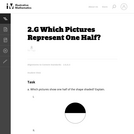Unrestricted Use
CC BY
Rating
0.0 stars

In this task students see different ways of partitioning a circle and a rectangle into two or more equal shares.

Subject:
Geometry
Mathematics
Ratios and Proportions
Material Type:
Activity/Lab
Provider:
Illustrative Mathematics
Provider Set:
Illustrative Mathematics
Author:
Illustrative Mathematics